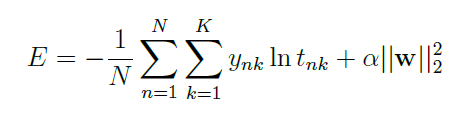How to add a L2 regularization term in my loss function

(Weimin023) #1

Hi, I’m a newcomer. I learned Pytorch for a short time and I like it so much.I’m going to compare the difference between with and without regularization, thus I want to custom two loss functions.

``````###OPTIMIZER
criterion = nn.CrossEntropyLoss()
optimizer = optim.SGD(net.parameters(), lr = LR, momentum = MOMENTUM)
``````

Can someone give me a further example? Thanks a lot!

BTW, I know that the latest version of TensorFlow can support dynamic graph.
But what is the difference of the dynamic graph between these two frameworks?

1 Like
(Sepehr Sameni) #2

set “weight_decay” parameter to a non zero value in your optimizer(sgd, adam, …)(it’s the alpha in your equation)
edit: I think it’s alpha times two actually

1 Like
(Weimin023) #3

I think I miss one row: def backward
Cuz ‘w’ is the weight. It is updated continuously by steps.
I just wonder if I need to do the grad-decent by myself?

(Sepehr Sameni) #4

oh, in that case, iterate over your parameters (for p in self.parameters()) and add (p**2).sum() to your loss

1 Like
(Weimin023) #5

Can you give me a simple example, ex: MSE
I’d like to know the mechanism of this custom class detaily.(Sepehr Sameni) #6

have you seen this?
there are two ways to handle backprop, doing it by hand or using the autograd package (and also a third way which is using both of them, by defining backward)
if you are using the autograd, and your modules are composed of standard operations, you can simply define your loss without the L2 regularizer and in the optimizer define the regularizer

``````class custom(nn.Module):
def __init__(self):
super().__init__()
def forward(self, x):
return x
net = custom()
optimizer = optim.SGD(net.parameters(), lr=0.01, weight_decay=0.01)
criterion = nn.MSELoss()
for batch in batches:
y = net(batch['x'])
loss = criterion(y, batch['y'])
loss.backward()
optimizer.step()

``````
2 Likes
(Weimin023) #7

Sure, I know this is custom neural network.

I use the 3 layers CNN net defined by myself with the nn.MSELoss() before. It’s autograd.
But now I want to compare the results if loss function with or without L2 regularization term.

If I use autograd nn.MSELoss(), I can not make sure if there is a regular term included or not.
p.s.:I checked that parameter ‘weight_decay’ in optim means “add a L2 regular term” to loss function.Furthermore, if I want to add a “L1” norm term in my loss function, I CANNOT USE THE autograd ?

(Sepehr Sameni) #8

no, you can always use autograd (even if your function does not have a derivative, you can use something else as derivative and go backward from there), what i meant was that when you have simple functions, there is no need to write backward() yourself

`````` loss = mse(pred, target)
l1 = 0
for p in net.parameters():
l1 = l1 + p.abs().sum()
loss = loss + lambda_l1 * l1
loss.backward()
optimizer.step()
``````
3 Likes
(Sepehr Sameni) #9

in general loss of a network has some terms, adding L2 term via optimizer class is really easy and there is no need to explicitly add this term (optimizer does it), so if you want to compare networks, you can simply tune weight_decay

3 Likes
#10

I want to follow an implementation of a Keras model in which only on some conv layers an l2 `kernel_regularizer` has been used. Now I have followed your implementation but am wondering if it suffices to filter for the names of the layers that I want to include my regularization on or not. I.e. along the lines of:

``````reg_lambda=0.01
l2_reg = 0
if isinstance(layer_names, list):
for W in self.model.named_parameters():
if "weight" in W:
layer_name = W.replace(".weight", "")
if layer_name in layer_names:
l2_reg = l2_reg + W.norm(2)
loss = loss + l2_reg * reg_lambda
loss.backward()
``````
1 Like
(Fs Z) #11

I have the same problem that use pytorch to achieve keras’s kernel_regularizer. can you share this part code that have worked .THX